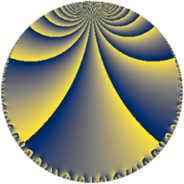# Properties

 Label 144.6.iLevel $144$ Weight $6$ Character orbit 144.i Rep. character $\chi_{144}(49,\cdot)$ Character field $\Q(\zeta_{3})$ Dimension $58$ Newform subspaces $6$ Sturm bound $144$ Trace bound $1$

# Related objects

## Defining parameters

 Level: $$N$$ $$=$$ $$144 = 2^{4} \cdot 3^{2}$$ Weight: $$k$$ $$=$$ $$6$$ Character orbit: $$[\chi]$$ $$=$$ 144.i (of order $$3$$ and degree $$2$$) Character conductor: $$\operatorname{cond}(\chi)$$ $$=$$ $$9$$ Character field: $$\Q(\zeta_{3})$$ Newform subspaces: $$6$$ Sturm bound: $$144$$ Trace bound: $$1$$ Distinguishing $$T_p$$: $$5$$

## Dimensions

The following table gives the dimensions of various subspaces of $$M_{6}(144, [\chi])$$.

Total New Old
Modular forms 252 62 190
Cusp forms 228 58 170
Eisenstein series 24 4 20

## Trace form

 $$58q + 2q^{3} - q^{5} + q^{7} + 20q^{9} + O(q^{10})$$ $$58q + 2q^{3} - q^{5} + q^{7} + 20q^{9} + 727q^{11} - q^{13} - 1675q^{15} + 1000q^{17} + 4q^{19} - 579q^{21} - 3173q^{23} - 15626q^{25} + 6176q^{27} - 3981q^{29} - 1625q^{31} - 4973q^{33} - 35646q^{35} - 4q^{37} + 13265q^{39} + 2901q^{41} - 13865q^{43} - 8677q^{45} - 37935q^{47} - 55224q^{49} - 40742q^{51} - 4q^{53} - 6246q^{55} + 3758q^{57} + 55033q^{59} - q^{61} - 75807q^{63} - 5743q^{65} + q^{67} + 53005q^{69} + 23672q^{71} - 53632q^{73} + 57646q^{75} + 24885q^{77} + q^{79} - 106708q^{81} + 41693q^{83} - 3126q^{85} + 211455q^{87} + 207948q^{89} - 80758q^{91} + 164837q^{93} + 61748q^{95} - 58147q^{97} + 31729q^{99} + O(q^{100})$$

## Decomposition of $$S_{6}^{\mathrm{new}}(144, [\chi])$$ into newform subspaces

Label Dim. $$A$$ Field CM Traces $q$-expansion
$$a_2$$ $$a_3$$ $$a_5$$ $$a_7$$
144.6.i.a $$4$$ $$23.095$$ $$\Q(\sqrt{-2}, \sqrt{-3})$$ None $$0$$ $$0$$ $$-54$$ $$-74$$ $$q+(-3+6\beta _{1}-\beta _{3})q^{3}+(-3^{3}\beta _{1}+2\beta _{2}+\cdots)q^{5}+\cdots$$
144.6.i.b $$6$$ $$23.095$$ 6.0.$$\cdots$$.3 None $$0$$ $$-9$$ $$-54$$ $$132$$ $$q+(-1-\beta _{1}-\beta _{2}+\beta _{3})q^{3}+(-20+\cdots)q^{5}+\cdots$$
144.6.i.c $$8$$ $$23.095$$ $$\mathbb{Q}[x]/(x^{8} + \cdots)$$ None $$0$$ $$12$$ $$78$$ $$-28$$ $$q+(3-3\beta _{1}+\beta _{4})q^{3}+(20\beta _{1}-2\beta _{2}+\cdots)q^{5}+\cdots$$
144.6.i.d $$10$$ $$23.095$$ $$\mathbb{Q}[x]/(x^{10} + \cdots)$$ None $$0$$ $$-12$$ $$-21$$ $$-29$$ $$q+(-2-2\beta _{1}+\beta _{3}+\beta _{4})q^{3}+(4\beta _{1}+\cdots)q^{5}+\cdots$$
144.6.i.e $$14$$ $$23.095$$ $$\mathbb{Q}[x]/(x^{14} - \cdots)$$ None $$0$$ $$0$$ $$25$$ $$-93$$ $$q+(-1+\beta _{2}+\beta _{4})q^{3}+(3-3\beta _{2}-\beta _{3}+\cdots)q^{5}+\cdots$$
144.6.i.f $$16$$ $$23.095$$ $$\mathbb{Q}[x]/(x^{16} + \cdots)$$ None $$0$$ $$11$$ $$25$$ $$93$$ $$q+(1-\beta _{1}-\beta _{6})q^{3}+(\beta _{2}+3\beta _{3}+\beta _{9}+\cdots)q^{5}+\cdots$$

## Decomposition of $$S_{6}^{\mathrm{old}}(144, [\chi])$$ into lower level spaces

$$S_{6}^{\mathrm{old}}(144, [\chi]) \cong$$ $$S_{6}^{\mathrm{new}}(9, [\chi])$$$$^{\oplus 5}$$$$\oplus$$$$S_{6}^{\mathrm{new}}(18, [\chi])$$$$^{\oplus 4}$$$$\oplus$$$$S_{6}^{\mathrm{new}}(36, [\chi])$$$$^{\oplus 3}$$$$\oplus$$$$S_{6}^{\mathrm{new}}(72, [\chi])$$$$^{\oplus 2}$$